### Home > A2C > Chapter Ch12 > Lesson 12.1.4 > Problem12-66

12-66.
1. Use algebra to demonstrate that each pair of expressions in parts (a) and (b) below are equivalent. Homework Help ✎

1.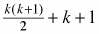and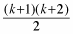2.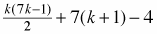and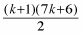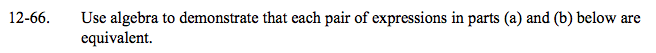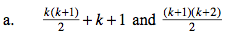Simplify and find a common denominator in the first expression:

$\frac{k^2+k+2k+2}{2}$

Now combine like terms and factor.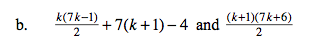Use the same method as in part (a).